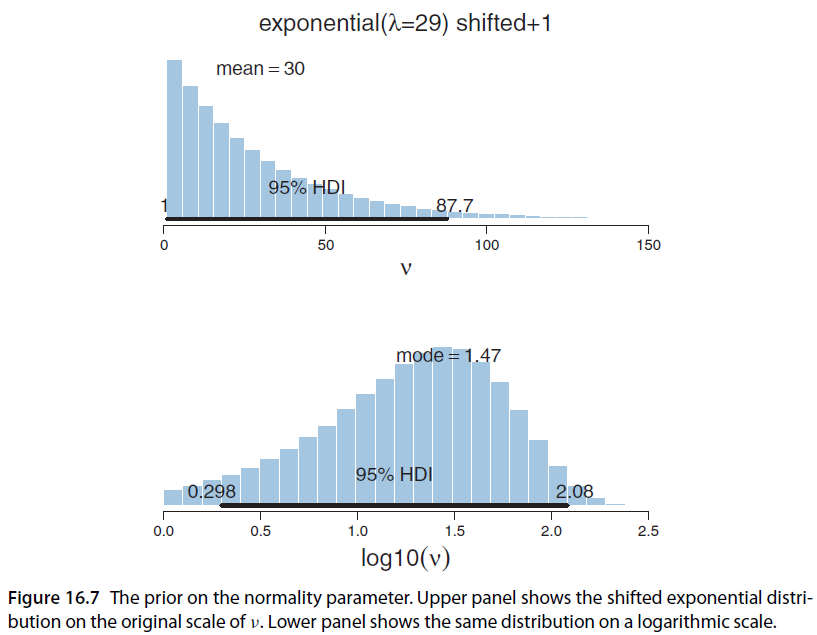## Wednesday, December 2, 2015

### Prior on df (normality) parameter in t distribution

Many models in DBDA use a t distribution for "robust" estimation of central tendency parameters. The idea is that the heavy tails of a t distribution can accommodate data points that would be outliers relative to a normal distribution, and therefore the estimates of the mean and standard deviation are not distorted by the outliers. Thus the estimates of the mean and standard deviation in the t distribution are "robust against outliers" relative to a normal distribution.

The parameter that controls the heaviness of the tails in a t distribution is called the degrees-of-freedom parameter, ν (nu). I prefer to call it the normality parameter because larger values make the distribution more normal, and the distribution is normal when ν is infinity. Values of ν larger than about 30.0 make the distribution roughly normal, and values of ν less than about 30.0 give the distribution noticeably heavier tails than a normal.

Here's the news: Past Self had learned, sometime long ago, that ν must be 1.0 or larger as an algebraic constraint in the definition of the t distribution. Past Self was wrong. In fact, ν must be 0.0 or larger. This issue was brought to my attention by Osvaldo Martin who asked me, in comments on a previous blog post, why I thought ν had to be greater than 1.0. Perhaps other people have asked me this question in the past, but somehow this is the first time I was prompted to double check my answer.

So what? Well, all the models involving a t distribution in the 1st and 2nd editions of DBDA had priors that restricted ν to be 1.0 or greater (see figure below). Fortunately, there is essentially no bad consequence whatsoever, because real data virtually never are distributed with such extreme outliers that an estimated value of ν<1 is needed. This lack of consequence is presumably why the error of Past Self has gone uncorrected so long.The prior used in DBDA2E. There's no need to shift it +1.

Despite the error being virtually harmless, I've changed all the programs so that the priors go from ν=0 up. This change simplifies the model specifications, too. Now the JAGS models simply specify

nu ~ dexp(1/30.0)

nu <- nuMinusOne + 1
nuMinusOne ~ dexp(1/29.0)

I've made this change in umpteen programs, including the Stan programs too. I've checked that all the JAGS programs still run fine, but I have not yet checked the Stan programs. Let me know if you encounter any problems. The programs are available at the book's web site.

The change also means that the diagrams of the models can be simplified, such as Figure 16.11 on p. 468. I'll leave that to your imagination.

1.Hi John,

Sorry to hijack this post but I thought I could post a question here anyway as it relates somehow to Bayesian data analysis. Do you have an opinion for maximum number of variables that could be processed simultaneously? I have a big cohort data containing over 50000 rows and 100 columns. One of the variables is dichotomous variable indicating if person had developed a certain disease during a time or not. I thought it would be nice to analyse all data to find out if there are any specific attributes that increase risk for the disease. Do you think it is reasonable to do this kind of analysis or is it just a waste of time?

If you consider this as waste of time how would you analyse this kind of cohort data?

2.Right, that's a bit irrelevant to this post, but here's an answer anyway :-)

You're proposing a logistic regression: The predicted variable is having/not having the disease, and there are several dozens of candidate predictors. There are also tens of thousands of data points.

In principle, there is no problem doing the analysis. But there are at least couple issues to think about in practice.

First, you are "fishing" for explanatory variables. You will want to put the regression coefficients under a hierarchical prior so that you get mutually-informed shrinkage of the regression coefficients. And even then, it would be good if you could confirm your findings in a separate, distinct data set.

Second, MCMC might crawl very slowly through such a large data set. Especially if the data+model happen to produce a posterior distribution with a lot of autocorrelation. I'd say to debug your model with a random subset of, say, 500 rows. Once you are sure that the model is working properly, increase the random subset to, say, 5000 rows and see what the autocorrelation is like and estimate how long it would take to do the full data set. Then enter all 50000 rows and let it run overnight.